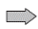Chapter 8.2, Problem 99E

Chapter
Section
Textbook Problem

# Finding an Error Find the fallacy in the following argument that 0 = 1 . d v = d xv = ∫ d x = x u = 1 xd u = − 1 x 2 d x 0 + ∫ d x x = ( 1 x ) ( x ) − ∫ ( − 1 x 2 ) ( x )   d x = 1 + ∫ d x x So, 0 = 1

To determine
The fallacy in the given argument that 0=1.

The argument is:

dv=dxv=dx=x

And

u=1xdu=1x2dx

Then,

0+dxx=(1x)(x)(1x2)(x)dx=1+1xdx

So, 0=1

Explanation

Any integrable function,

f(x)dx=f(

### Still sussing out bartleby?

Check out a sample textbook solution.

See a sample solution

#### The Solution to Your Study Problems

Bartleby provides explanations to thousands of textbook problems written by our experts, many with advanced degrees!

Get Started

#### In Exercises 2340, find the indicated limit. 25. limx3x

Applied Calculus for the Managerial, Life, and Social Sciences: A Brief Approach

#### In problems 17-20, indicate whether the two sets are equal. 19.

Mathematical Applications for the Management, Life, and Social Sciences

#### Multiply: (9)(1)

Elementary Technical Mathematics